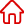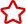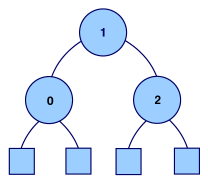﻿ 診斷Java代碼: Double Descent錯誤模式_關於JAVA設為首頁 加入收藏

C語言|JAVA編程
Python編程

ASP編程|PHP編程
JSP編程

MYSQL數據庫|SqlServer數據庫
Oracle數據庫|DB2數據庫
程式師世界 >> 編程語言 >> JAVA編程 >> 關於JAVA >> 診斷Java代碼: Double Descent錯誤模式

# 診斷Java代碼: Double Descent錯誤模式

Double Descent 錯誤模式

```abstract class Tree { } class Leaf extends Tree { 　 public static final Leaf ONLY = new Leaf(); } class Branch extends Tree { 　 public int value; 　 public Tree left; 　 public Tree right; 　 public Branch(int _value, Tree _left, Tree _right) { 　　 this.value = _value; 　　 this.left = _left; 　　 this.right = _right; 　 } }```

```// in class Tree: 　 public abstract boolean hasConsecutiveZeros(); 　 // in class Leaf: 　 public boolean hasConsecutiveZeros() { 　　 return false; 　 } 　 // in class Branch: 　 public boolean hasConsecutiveZeros() { 　　 boolean foundOnLeft = false; 　　 boolean foundOnRight = false; 　　 if (this.value == 0) { 　　　 foundOnLeft = this.left.value == 0; 　　　 foundOnRight = this.right.value == 0; 　　 } 　　 if (foundOnLeft || foundOnRight) { 　　　 return true; 　　 } 　　 else { 　　　 foundOnLeft = this.left.hasConsecutiveZeros(); 　　　 foundOnRight = this.right.hasConsecutiveZeros(); 　　　 return foundOnLeft || foundOnRight; 　　 } 　 }```

```public boolean hasConsecutiveZeros() { 　　 boolean foundOnLeft = false; 　　 boolean foundOnRight = false; 　　 if (this.value == 0) { 　　　 foundOnLeft = ((Branch)this.left).value == 0; 　　　 foundOnRight = ((Branch)this.right).value == 0; 　　 } 　　 if (foundOnLeft || foundOnRight) { 　　　 return true; 　　 } 　　 else { 　　　 foundOnLeft = this.left.hasConsecutiveZeros(); 　　　 foundOnRight = this.right.hasConsecutiveZeros(); 　　　 return foundOnLeft || foundOnRight; 　　 } 　 }``````if (! (this.left instanceof Leaf)) {　 　　　 // this.left instanceof Branch 　　　 foundOnLeft = ((Branch)this.left).value == 0; 　　 } 　　 if (! (this.right instanceof Leaf)) { 　　　 // this.right instanceof Branch 　　　 foundOnRight = ((Branch)this.right).value == 0; 　　 }```

```// in class Tree: 　 public abstract boolean valueIs(int n); 　 // in class Leaf: 　 public boolean valueIs(int n) { return false; } 　 // in class Branch: 　 public boolean valueIs(int n) { 　　 return value == n; 　 } 　 // in class Branch, method hasConsecutiveZeros 　 if (this.valueIs(0)) { 　　 foundOnLeft = this.left.valueIs(0); 　　 foundOnRight = this.right.valueIs(0); 　 }```

valueIs 通過封裝我們真正希望每個類單獨處理的內容：檢查類的一個實例是否包含給定的值，以避開所有這些問題。